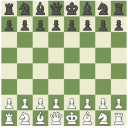QlikView App Dev

Discussion Board for collaboration related to QlikView App Development.

Announcements
QlikWorld 2022, LIVE in Denver CO., May 16-19, 2022. REGISTER NOW TO RECEIVE EARLY BIRD PRICING
cancel
Showing results for
Did you mean:Creator

Using a string as a fieldname

Hi,

I would like to use a constructed string, for example:

'TID_MONTH_ABR_' & Num(Month(WORK_DATE), '00')

as a field name in a function, for example:

=Only(TID_MONTH_ABR_01)

=Only(TID_MONTH_ABR_02)

...

=Only(TID_MONTH_ABR_12)

Thus:

= Only('TID_MONTH_ABR_' & Num(Month(MONTHDATE), '00'))

I use these TID_ fields in a multilingual environment so the TID-field give me the correct translations in de selected language

So how can I use the string as a field name in a function. Do I put \$() or {} or  ... around it?

R.W.

1 Solution

Accepted SolutionsMVP

May be with dollar sign expansion

=Only(\$(='TID_MONTH_ABR_' & Num(Month(MONTHDATE), '00')))

2 RepliesMVP

May be with dollar sign expansion

=Only(\$(='TID_MONTH_ABR_' & Num(Month(MONTHDATE), '00')))Employee

I'm wondering whether this approach can work for total <field>?

It works fine: if within <> I write MyField , the formula then looks the following way:  sum(VALUE) / sum(total <MyField>  VALUE)

PROBLEM: MyField variates due to the drilling down, so that it can be MyField1,MyField2,MyField3...

It does not work: if within <> I write MyVariable containing the needed field, the formula then looks the following way:  sum(VALUE) / sum(total <vMyVariable> VALUE)

If vMyVariable returns me a string '' which should be interpreted as a field, then according to this post I need something like only(\$(=\$(vMyVariable))) to put it within <> so that my formula looks like: sum(VALUE) / sum(total <only(\$(=\$(vMyVariable))) > VALUE). Unfortunately this does not work for me.

Any ideas/suggestions are very much appreciated.Community Browser